## Tech Notes

Common Light Measurement Terms
 The purpose of this document is to give a basic understanding of the common measuring Techniques and Terms that are used by the lighting industry. Candela: The unit of luminous intensity. One candela is defined as the luminous intensity of 1/600,000 square meter of projected area of a blackbody radiator operating at the temperature of solidification of platinum under pressure of 101,325 Newtons per square meter. (see Figure 1) Footcandle: A footcandle is a measure of light intensity. A footcandle is defined as the amount of light received by 1 square foot of a surface that is 1 foot from a point source of light equivalent to one candle of a certain type. (see Figure 2) End Footcandle: End Footcandle measurements are based on the focused light beam only. The spherical energy or surrounding light output is not captured by or reflected back to the surface of the footcandle light meter. End footcandle is the focal light beam measurement from point A to point B at one-foot distance. (see Figure 3) Lumen: A unit of light flow or luminous flux. The lumen rating of a lamp is a measure of the total light output of the lamp. The most common measurement of light output  (or luminous flux) is the lumen. Light sources are labeled with an output rating in lumens. For example, a R30 65-Watt indoor flood lamp may have a rating of 750 lumens. Similarly, a light fixture's output can be expressed in lumens.  As lamps and fixtures age and become dirty, their lumen output decreases  (i.e., lumen depreciation occurs). Most lamp ratings are based on initial lumens (i.e., when lamp is new). (see Figure 4) End Lumens: End Lumens measurements are based on a spot of light only. The spherical energy or surrounding light output is not captured by or reflected back to the surface of the lumen light meter. End lumens is the light measurement from point A to point B at one-foot distance. (see Figure 5) Luminance: Luminous Flux (light output). This is the quantity of light that leaves the lamp, measured in lumens (lm). Lamps are rated in both initial and mean lumens. Initial lumens indicate how much light is produced once the lamp has stabilized; for fluorescent and high-intensity discharge (HID) lamps, this is typically 100 hours. Mean lumens indicate the average light output over the lamp's rated life, which reflects the gradual deterioration of performance due to the rigors of continued operation; for fluorescent lamps, this is usually determined at 40% of rated life. Luminous (Light Level): This is the amount of light measured on the work plane in the lighted space. The work plane is an imaginary horizontal, tilted or vertical line where the most important tasks in the space are performed. Measured in footcandles (fc or lux in metric), light levels are either calculated, or in existing spaces, measured with a light meter. A footcandle is actually one lumen of light density per square foot; one lux is one lumen per square meter. Like lumens, footcandles can be produced as either initial or maintained quantities. Work Plane: The level at which work is done where illuminance is specified and measured. For office applications, this is typically a horizontal plane 30 inches above the floor (e.g., desk height). Beam Lumens: The total flux in that region of space where the intensity exceeds 50 percent of the maximum intensity. Field Lumens: The total flux in that region of space where the intensity exceeds ten percent of the maximum intensity. Lux: The metric unit of measure for illuminance of a surface. One lux is equal to one lumen per square meter. One lux equals 0.0929 footcandles. Light Level: Light intensity measured on a plane at a specific location is called illuminance. Illuminance is measured in footcandles, which are workplane lumens per square foot. You can measure illuminance using a light meter located on the work surface where tasks are performed. Using simple arithmetic and manufacturers' photometric data, you can predict illuminance for a defined space. (Lux is the metric unit for illuminance, measured in lumens per square meter. To convert footcandles to lux, multiply footcandles by 10.76). Efficacy: A measure of the luminous efficiency of a radiant flux, expressed in lumens per watt as the quotient of the total luminous flux by the total flux. For daylighting, this is the quotient of visible flux incident on a surface to radiant flux on that surface. For electric sources, this is the quotient of the total luminous flux emitted by the total lamp power input. Efficacy of a Light Source: The total light output of a light source divided by the total power input. Efficacy is expressed in lumens per Watt. Watt: The unit of measuring electrical power. Watts does not relate to the light output level. It defines the rate of energy consumption by an electrical device when it is in operation. The energy cost of operating an electrical device is calculated as its wattage time in hours of use. In single-phase circuits, it is related to volts and amps by the formula: Volts x Amps x Power Factor (PF) = Watts. (Note: For AC circuits, PF must be included). Kilowatt Hour (kWh) Formula: The measure of electrical energy from which electricity billing is determined. For example, a 100-Watt bulb operated for 1000 hours would consume 100 kilowatt hours (100 Watts x 1000 hours = 100 kWh). At a billing rate of \$0.10/kWh, this bulb would cost \$10.00 (100 kWh x \$0.10/kWh) to operate over 1000 hours. Source: Light Resource.com, Light Research Center, Light Board, IES Lighting Handbook, 5th Edition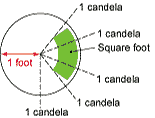Figure 1. Candela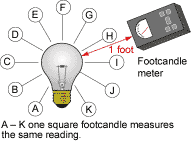Figure 2. Footcandle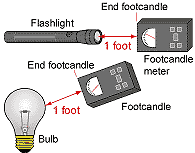Figure 3. End Footcandle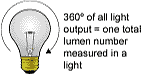Figure 4. Lumens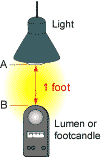Figure 5. End Lumens